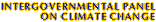Climate Change 2001: Working Group I: The Scientific BasisGet Javascript Other reports in this collection

### 6.3.2 Methane and Nitrous Oxide

The SAR reported that several studies found a higher forcing due to CH4 than IPCC (1990), up to 20%; however the recommendation was to use the same value as in IPCC (1990). The higher radiative forcing estimates were obtained using band models. Recent calculations using LBL and band models confirm these results (Lelieveld et al., 1998; Minschwaner et al., 1998; Jain et al., 2000). Using two band models, Myhre et al. (1998b) found the computed radiative forcing to differ by almost 10%. This was attributed to difficulties in the treatment of CH4 in band models since, given its present abundance, the CH4 absorption lies between the weak line and the strong line limits (Ramanathan et al., 1987). After updating for a small increase in concentration since the SAR, the radiative forcing due to CH4 is 0.48 Wm-2 since pre-industrial times. This estimate for forcing due to CH4 is only for the direct effect of CH4; for radiative forcing of the indirect effect of CH4, see Sections 6.5 and 6.6.

The problem mentioned above with the band models for CH4 does not occur to the same degree in the case of N2O, given the latters present concentrations. Three recent studies, Myhre et al. (1998b) (two models), Minschwaner et al. (1998) (one model), and Jain et al. (2000) (one model), calculated lower radiative forcing for N2O than reported in previous IPCC assessments, viz., 0.13, 0.12, 0.11, and 0.12 Wm-2, respectively, compared to 0.14 Wm-2 in the SAR. For N2O, effects of change in spectroscopic data, stratospheric adjustment, and decay of the mixing ratio in the stratosphere are all found to be small effects. However, effects of clouds and different radiation schemes are potential sources for the difference between the newer estimates and the SAR. A value of 0.15 Wm-2 is now suggested for the radiative forcing due to N2O, taking into account an increase in the concentration since the SAR, together with a smaller pre-industrial concentration than assumed in IPCC (1996a; Table 2.2) (see Chapter 4).